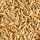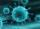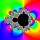# Piano

If Suzan practicing 10 minutes at Monday; every other day she wants to practice 2 times as much as the previous day, how many hours and minutes will have to practice on Friday?

Result

x = 2:40 (hh:mm)#### Solution:We would be pleased if you find an error in the word problem, spelling mistakes, or inaccuracies and send it to us. Thank you!## Next similar math problems:

• LineIt is true that the lines that do not intersect are parallel?
• Reference angleFind the reference angle of each angle:
• ChordsHow many 4-tones chords (chord = at the same time sounding different tones) is possible to play within 7 tones?
• GP - 8 itemsDetermine the first eight members of a geometric progression if a9=512, q=2
• Geometric progression 48,4√2,4,2√2
• Six termsFind the first six terms of the sequence a1 = -3, an = 2 * an-1
• Five harvestsIn the seed company, they know that, out of 100 grains of a new variety, they get an average of 2000 grains after harvest. Approximately how many grains do they get out of 100 grains after five harvests?
• Geometric progression 2There is geometric sequence with a1=5.7 and quotient q=-2.5. Calculate a17.
• Terms of GPWhat is the 6th term of the GP 9, 81, 729,. .. ?
• CoefficientDetermine the coefficient of this sequence: 7.2; 2.4; 0.8
• Five membersWrite first 5 members geometric sequence and determine whether it is increasing or decreasing: a1 = 3 q = -2
• Tenth memberCalculate the tenth member of geometric sequence when given: a1=1/2 and q=2
• Three workshopsThere are 2743 people working in three workshops. In the second workshop works 140 people more than in the first and in third works 4.2 times more than the second one. How many people work in each workshop?
• Missing term 2What is the missing term for the Geometric Progression (GP) 3, 15, 75,__, 1875?
• VirusWe have a virus that lives one hour. Every half hour produce two child viruses. What will be the living population of the virus after 3.5 hours?
• Find next memberFind x if the numbers from a GP 7, 49, x.
• Geometric progressionFill 4 numbers between 4 and -12500 to form geometric progression.